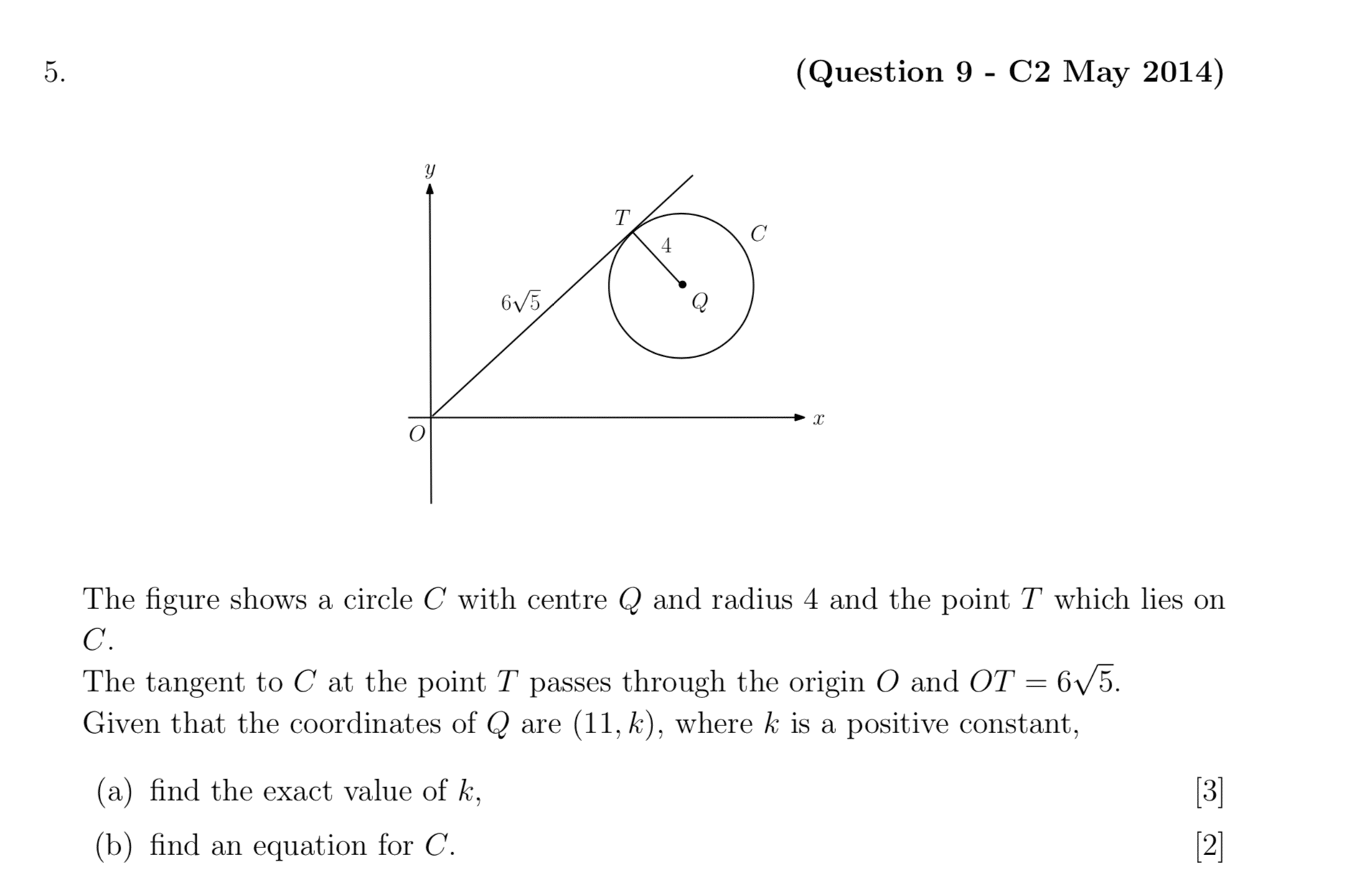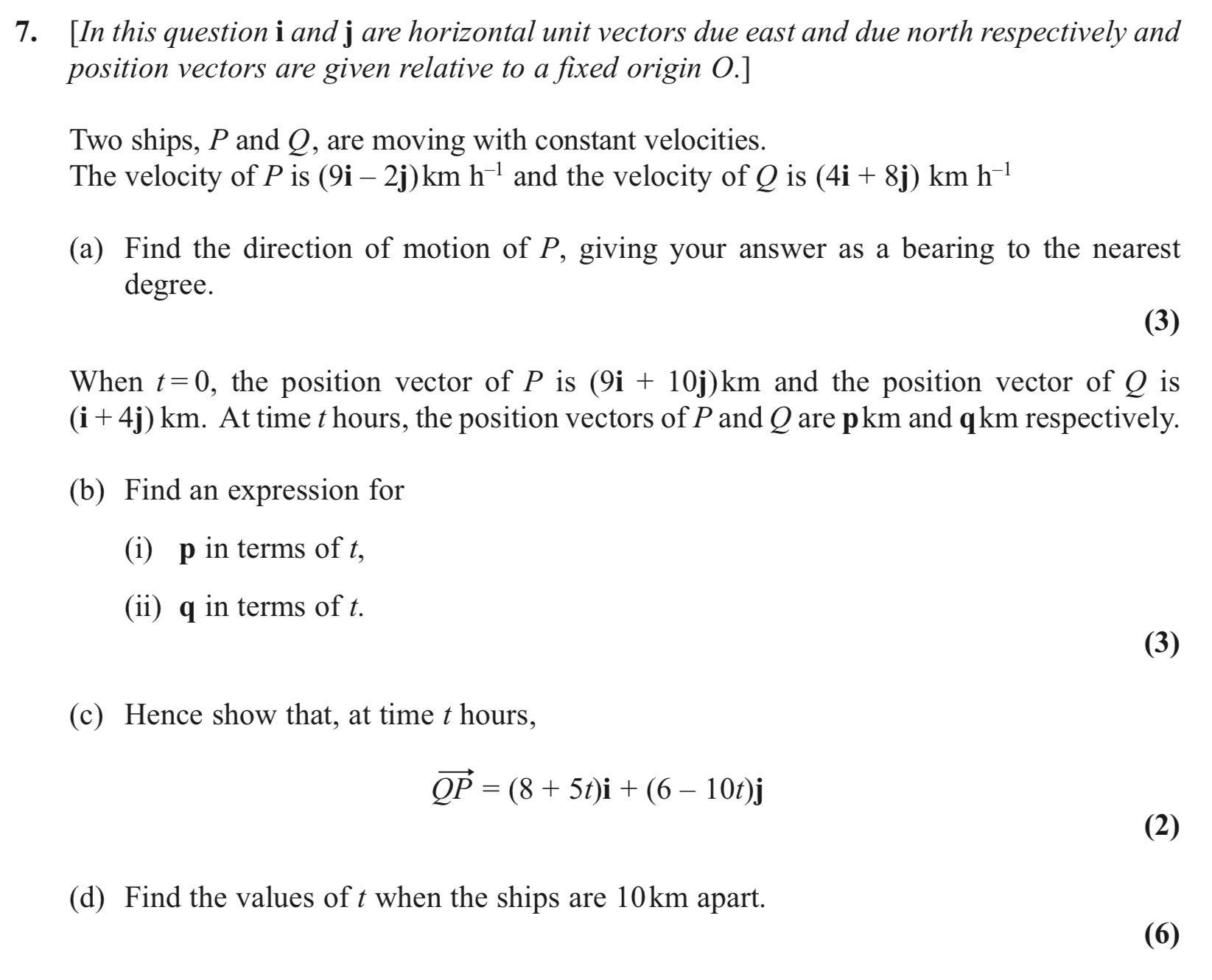## Question of the Week – Week 51

The diagram shows the curve$y=x^2$ and the straight line$y=0.5x+2$. Find the shaded area shown in purple to 3 decimal places.## Question of the Week – Week 50## Question of the Week – Week 49

Show that the difference between the squares of two consecutive odd numbers is always a multiple of 8.

## Question of the Week – Week 48## Question of the Week – Week 47

Solve$2^{2x+4}=9\left(4^{0.5x}\right)$.

## Question of the Week – Week 46

Given that$V=17000e^{-0.25t}+2000e^{-0.5t}+500$

find the value of t when V=9500.

## Question of the Week – Week 45

Prove that the difference between a whole number and its cube is a multiple of 6.

## Question of the Week – Week 44

The following table shows the prices in millions of pounds of some houses in a given postcode:

 House Price, P (£m) Frequency$0.2\leq P\hspace{2pt}\textless\hspace{2pt} 0.3$$21$$0.3\leq P\hspace{2pt}\textless\hspace{2pt} 0.4$$37$$0.4\leq P\hspace{2pt}\textless\hspace{2pt} 0.5$$24$$0.5\leq P\hspace{2pt}\textless \hspace{2pt}0.6$$11$$0.6\leq P\hspace{2pt}\textless\hspace{2pt} 0.7$$7$

Find an estimate for the interquartile range for this data.

## Question of the Week – Week 43

Find, in terms of m, the coordinates of the point (1,0) after being reflected in the line$y=mx$.

## Question of the Week – Week 42

Find rational numbers x and y such that$4^x\times 3^y=24$.

## Question of the Week – Week 41

Solve$\frac{1}{3^{x}-1}+3^{1-x}=1.5$

## Question of the Week – Week 40

The points (0,6), (9,0), (0,-6) and (-k,0) lie on the circumference of a circle. Find the value of k.

## Question of the Week – Week 39

The diagram shows the curve with equation$y=-x(x-2)(x+1)$. Find the area shaded shown in green.## Question of the Week – Week 38

Given that$f(x)=x^{\frac{1}{2}}+9x^{-\frac{1}{2}}$ (and$x\ne 0$), find the values of x for which f(x) is a decreasing function.

## Question of the Week – Week 37

Prove that$\frac{\cos^4(\theta)-\sin^4(\theta)}{\cos^2(\theta)}\equiv 1-\tan^2{\theta}$.

## Question of the Week – Week 36

The points A and B have position vectors$3{\bf i}-4{\bf j}$ and$2{\bf j}-7{\bf i}$ respectively. The point P splits the line vector$\overrightarrow{AB}$ in the ratio 2:3. Find the position vector$\overrightarrow{OP}$.

## Question of the Week – Week 35

A ship leaves a port and heads to its destination for 65 km on a bearing of$071^\circ$ before receiving a distress signal. The distress signal is sent from a vessel with a position that is 54km away from and at a bearing of$122^\circ$ relative to the port. Calculate the distance between the vessel in distress and the ship.

## Question of the Week – Week 34From C2 Edexcel Paper June 2013.

## Question of the Week – Week 33From C2 Edexcel Paper May 2014.

## Question of the Week – Week 32

Point P with x-coordinate 0.5 lies on the curve with equation$y=2x^2$. The normal to the curve at point P intersects the curve at points P and Q. Find the coordinates of Q.

## Question of the Week – Week 31From Edexcel C2 June 2017 exam.

## Question of the Week – Week 30From Edexcel C2 January 2010 exam.